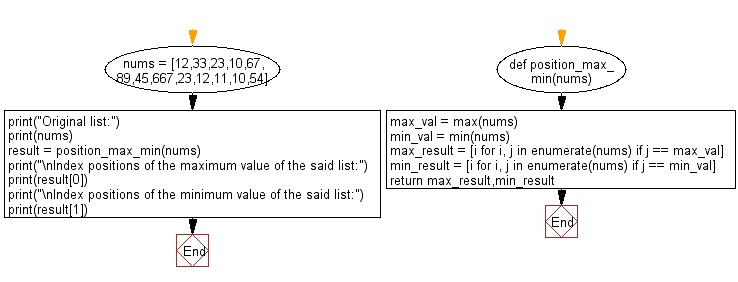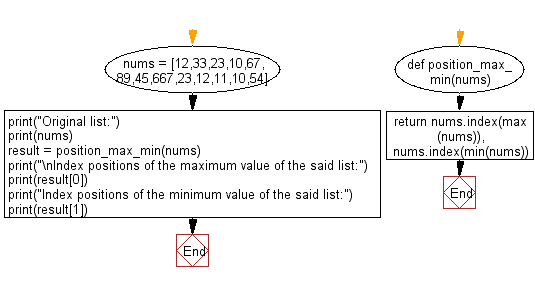﻿ Python: Find all index positions of the maximum and minimum values in a given list - w3resource# Python: Find all index positions of the maximum and minimum values in a given list

## Python List: Exercise - 132 with Solution

Write a Python program to find all index positions of the maximum and minimum values in a given list of numbers.

Sample Solution-1:

Python Code:

``````def position_max_min(nums):
max_val = max(nums)
min_val = min(nums)
max_result = [i for i, j in enumerate(nums) if j == max_val]
min_result = [i for i, j in enumerate(nums) if j == min_val]
return max_result,min_result

nums = [12,33,23,10,67,89,45,667,23,12,11,10,54]
print("Original list:")
print(nums)
result = position_max_min(nums)
print("\nIndex positions of the maximum value of the said list:")
print(result)
print("\nIndex positions of the minimum value of the said list:")
print(result)
```
```

Sample Output:

```Original list:
[12, 33, 23, 10, 67, 89, 45, 667, 23, 12, 11, 10, 54]

Index positions of the maximum value of the said list:


Index positions of the minimum value of the said list:
[3, 11]
```

Flowchart:## Visualize Python code execution:

The following tool visualize what the computer is doing step-by-step as it executes the said program:

Sample Solution-2:

• Use max() and list.index() to get the maximum value in the list and return its index.
• Use min() and list.index() to get the minimum value in the list and return its index.

Python Code:

``````def position_max_min(nums):
return nums.index(max(nums)), nums.index(min(nums))
nums = [12,33,23,10,67,89,45,667,23,12,11,10,54]
print("Original list:")
print(nums)
result = position_max_min(nums)
print("\nIndex positions of the maximum value of the said list:")
print(result)
print("Index positions of the minimum value of the said list:")
print(result)
```
```

Sample Output:

```Original list:
[12, 33, 23, 10, 67, 89, 45, 667, 23, 12, 11, 10, 54]

Index positions of the maximum value of the said list:
7
Index positions of the minimum value of the said list:
3
```

Flowchart:## Visualize Python code execution:

The following tool visualize what the computer is doing step-by-step as it executes the said program:

Python Code Editor:

Have another way to solve this solution? Contribute your code (and comments) through Disqus.

What is the difficulty level of this exercise?

Test your Python skills with w3resource's quiz

﻿

## Python: Tips of the Day

```print(2_000_000)
```2000000